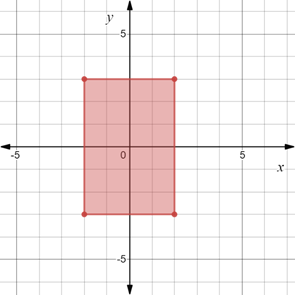# To graph : The region provided by the set { ( x , y ) | | x | ≤ 2 and | y | ≤ 3 } .### Precalculus: Mathematics for Calcu...

6th Edition
Stewart + 5 others
Publisher: Cengage Learning
ISBN: 9780840068071### Precalculus: Mathematics for Calcu...

6th Edition
Stewart + 5 others
Publisher: Cengage Learning
ISBN: 9780840068071

#### Solutions

Chapter 1.8, Problem 32E
To determine

## To graph: The region provided by the set {(x,y)||x|≤2 and |y|≤3} .

Expert Solution

### Explanation of Solution

Given information:

The set, {(x,y)||x|2 and |y|3} .

Graph:

The graph of the region provided by the set {(x,y)||x|2 and |y|3} is sketched in the coordinate plane.

Recall that |x|a is equivalent to axa .

Therefore, |y|3 is equivalent to 3y3 .

And |x|2 is equivalent to 2x2 .

Construct horizontal lines y=3 and y=3 and shaded the region between it.

Construct vertical lines x=2 and x=2 and shaded the region between it.

Now, shade the common region that belong to both the conditions stated above.

The region obtained is provided below,Interpretation:

The region provided by the set {(x,y)||x|2 and |y|3} is shaded region. The solid vertical line at x=2 and x=2 also horizontal lines y=3 and y=3 denote that they are included in the region. The region consists of all those points whose y-coordinate lie between y=3 and y=3 and x-coordinate lie between x=2 and x=2 .

### Have a homework question?

Subscribe to bartleby learn! Ask subject matter experts 30 homework questions each month. Plus, you’ll have access to millions of step-by-step textbook answers!